# S7E3 - Hydrogen Emission Spectrum and the Bohr Model of Hydrogen

Before we proceed, know that a "continuous spectrum" is a rainbow-like spectrum that occurs when a prism diffracts ("splits") white light.

## The Atomic Emission Spectrum for Hydrogen

When a hydrogen atom absorbs energy (hv), its single electron becomes excited and moves to a higher energy state.

When the excited electron later falls back or "relaxes" back down to its ground state, the electron releases the absorbed energy by emitting light of various wavelengths.

➞  what results is the line emission spectrum for hydrogen.

Here's a visual of 3 types of relaxations of an electron from 3 different excited states (n = 3, n = 4, n = 5), to the n = 2 state: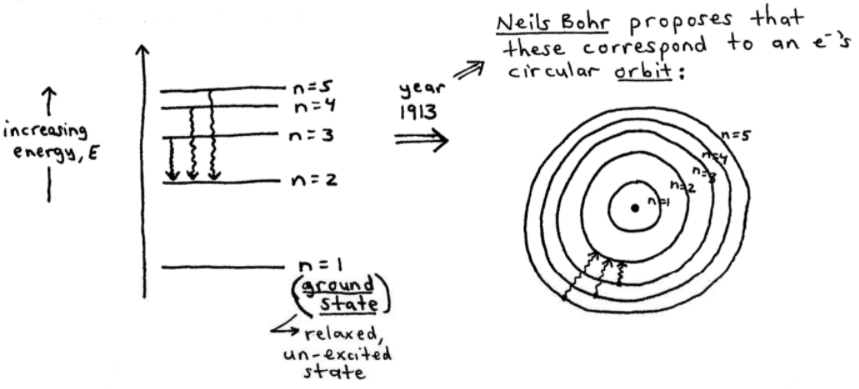In 1913, Neils Bohr proposed that the 3 relaxations and the energy levels (n) correspond to an electron's circular orbit.

Additionally, these 3 relaxations correspond to 3 of the 4 lines in the line spectrum for hydrogen:

## Emission Spectrum for Hydrogen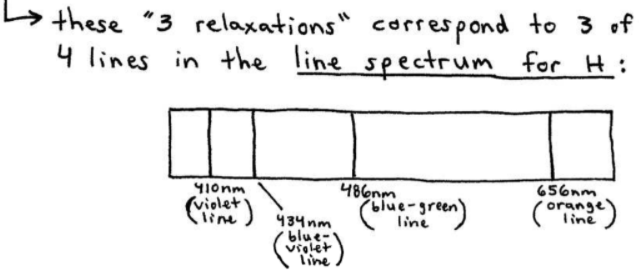## Bohr Model for Hydrogen

In Bohr's circular orbit model, the electron cannot be found "just anywhere," but instead only in those n = 1, 2, 3, ... energy-level orbits.

➞  mathematically, these quantized energy levels available to hydrogen's electron are given by the Rydberg equation...

### The Rydberg Equation----------

ex:  Calculate the energy required to excite the hydrogen electron from level n = 1 to level n = 2.  Also, calculate the wavelength of light that must be absorbed by a hydrogen atom in its ground state to reach this excited state.
_________
answer:Now, calculating the wavelength (λ) gives us 1.216 x 10-7 m, as shown here: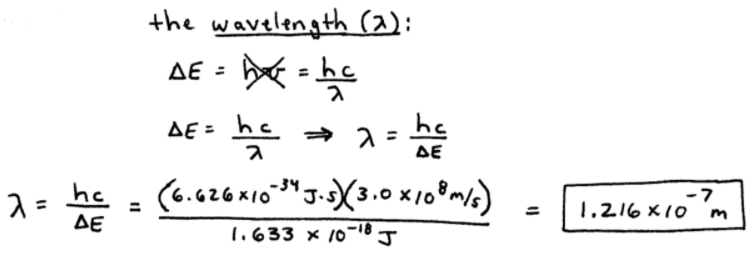==========

NOTE - In the last example, the first equation used is often re-written as: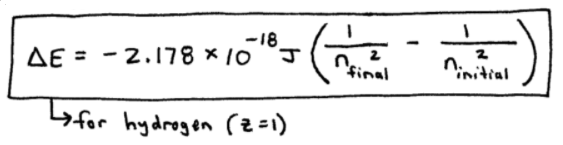----------

ex:  Calculate the energy required to remove the electron from a hydrogen atom in its ground state.
_________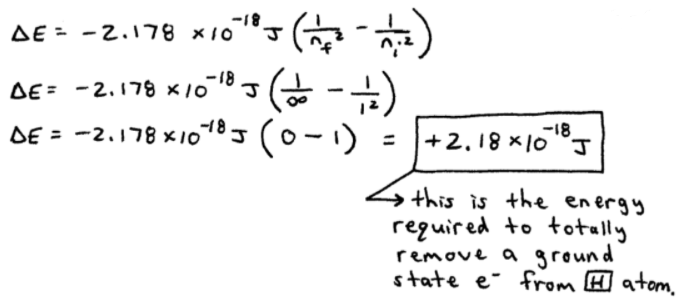==========